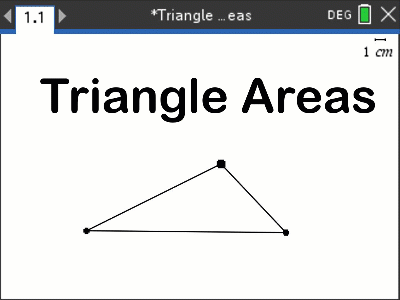# Activities

•• ##### AuthorMiddle

50 Minutes

• ##### Device
•TI-Nspire™ CX
•TI-Nspire™ CX CAS
• ##### Software

TI-Nspire™ CAS
TI-Nspire™

5.0

## Triangle Area#### Activity Overview

Draw a triangle and measure the area, now lock the area so that it cannot change. What forms might the triangle take now? Students explore the family of triangles created when the area cannot vary. Based on their findings, students work towards the formula for the area of a triangle.

#### Objectives

Students determine the formula for the area of a triangle based on observations.

#### Vocabulary

• Triangle
• Vertex
• Variable
• Base (triangle)

#### About the Lesson

Students create a free form triangle and measure the area. After some exploration, students lock the area of the triangle so that it cannot be changed. What forms can the triangle take on now? Trace on of the vertices and explain your observations. Which dimensions are variable? Which ones are invariant? Students draw conclusions about the formula for the area of a triangle based on their explorations and observations.